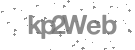# How to draw an arc with a specific arc length?

There are some methods that seem to allow you define an arc length. For example; start, center, length. But this is defining the chord length, not the arch length.

Calculating the angle using simple mathematics calculation, comparing the arch radius and arch length. After you get the angle value, then you can draw it. But it is not easy!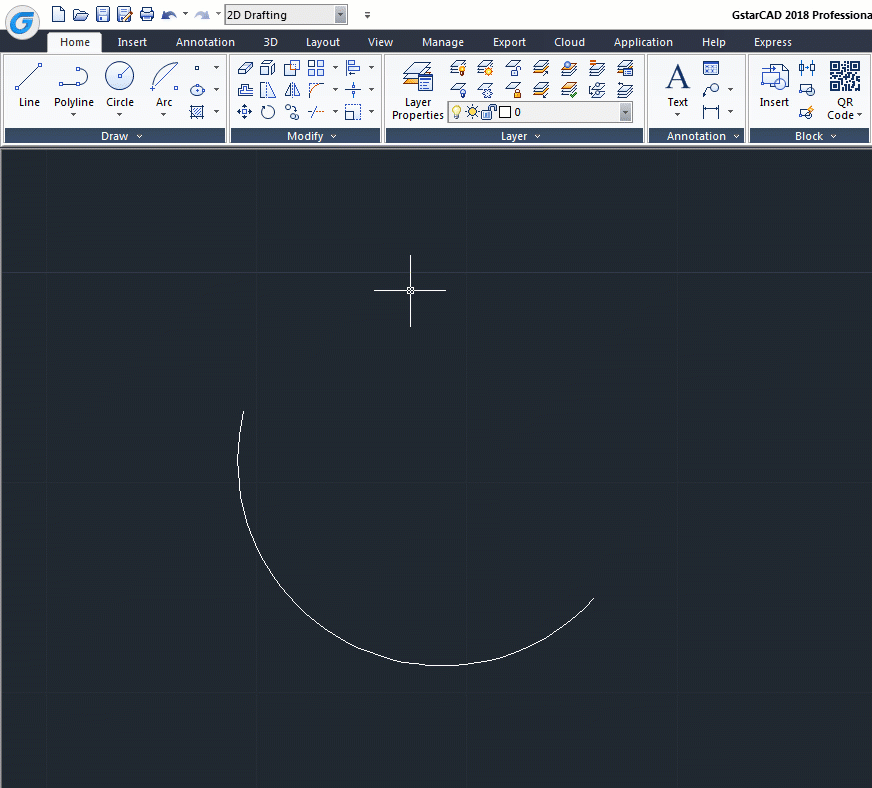A better solution is drawing the arch, then modify it using LENGTHEN command. Lengthen command allows you to define the object total length, including an arch. Select the total length option, then type the distance you want.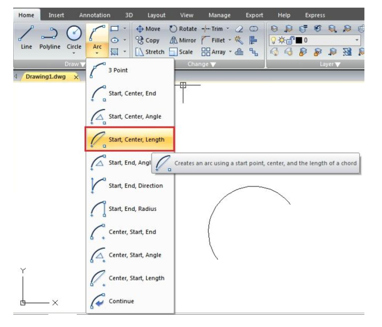A better solution is drawing the arch, then modify it using LENGTHEN command. Lengthen command allows you to define the object total length, including an arch. Select the total lengthoption, then type the distance you want.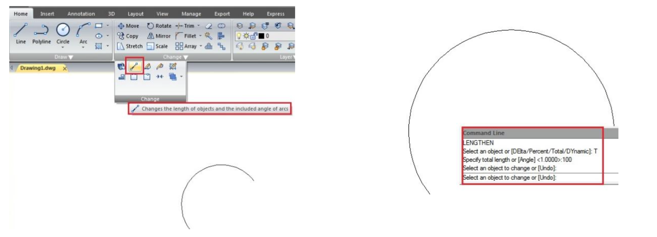Now if you try to inquiry or see the arc properties, you will see the arch has the exact length I wanted.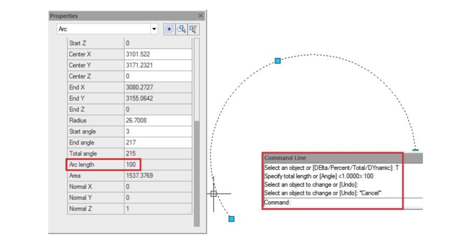#### Related tutorial video: